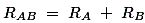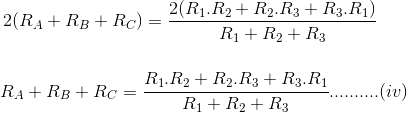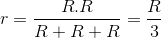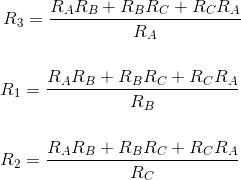# Simple Steps to Solve Delta to Star Conversion Formula (2023)We can connect the three branches of the electrical network in different ways. But this system commonly seen in the market is either a star or a delta. The three branches of a delta connection are connected to each other in a closed loop, so the form drawing is known as a delta connection.

When the terminals of these three branches are connected to a common point and form a Y shape in a star connection, it is known as a star connection. But these star and delta connections can be easily changed from one form to another. Delta-to-star or star-to-delta transformation becomes inevitable. In today’s article, you will see why a star can be converted into a delta and a delta into a star.

In This Post

## Delta To Star Conversion:Delta or triangle loop replacement by identical star connection is known as delta-star transformation. If a barrier is measured between any pair of barrier lines then the two connections are equal to or equal to each other.

This means that the value of impedance remains the same whether it is measured between any two pairs of lines whether it is connected between delta lines or its equivalent star connected between those lines.

The triangles are named A, B, and C respectively as shown in the figure. The resistance between points A and B is named R1 just as the resistance between points B and C is named R2 and the resistance between A and C is named R3.As shown in the figure the star connection is connected to a point named A, B, and C. respectively. In this star system the resistances RA, RB, and RC are connected to A, B, and C respectively. Now if we measure the resistance value between points A and B we get this.Since the star and delta systems are similar, the resistance measured between terminals A and B of these two systems must be the same.Just as the resistance between A and B is the same, so is the resistance between B and C.Similarly, the resistance between points C and A will be the same.Adding the equations we get (I), (II), and (III),Subtracting Equations (I), (II), and (III) from Equation (IV),The relation of delta-star change can be shown as follows:

The same star resistance connected to the given terminal is equal to the product of two delta resistances connected to the same terminal divided by the sum of the resistances connected to the delta.

If the delta-connected system has the same resistance R on its three sides, then the same star resistance will be r.### Star To Delta Conversion:

For the conversion from star to delta we just multiply (v), (VI) and (VI), (VII) and (VI), (V) which is (v) VI (VI) + (VI) (VII ) Is done)) + (VII) × (V) we get,Now we get the equation of dividing by Equations (V), (VI), and Equations (VIII) separately,Like this post? Could you share it with your friends?1.